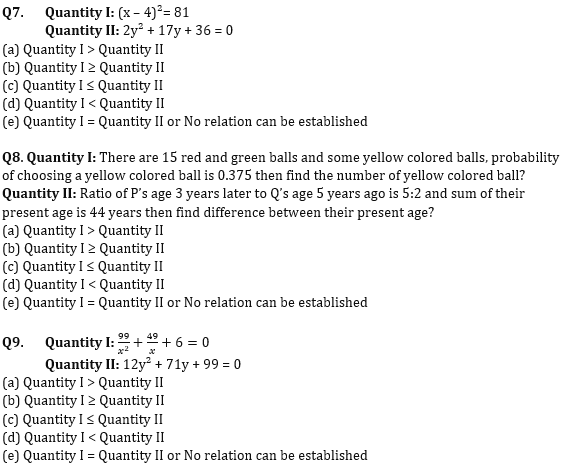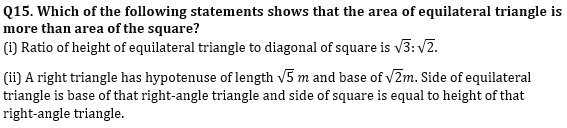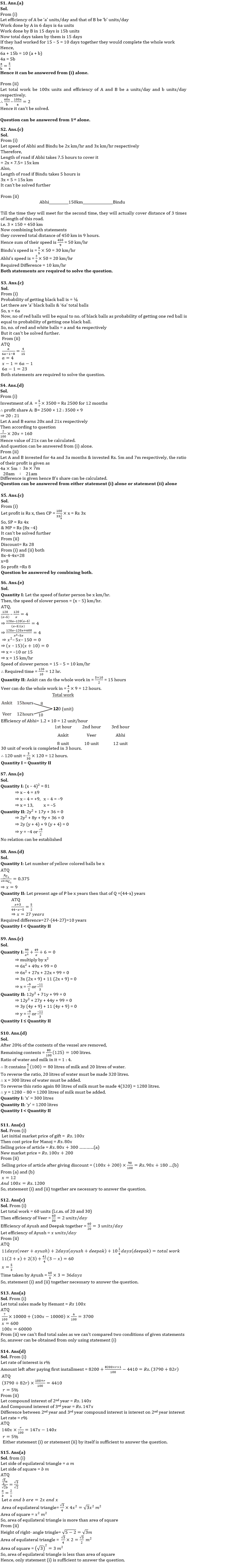Latest Banking jobs   »   Quantitative Aptitude Quiz For IBPS SO...

# Quantitative Aptitude Quiz For IBPS SO Prelims 2022- 28th November

Directions (1-5): The following questions are accompanied by two statements (i) and (ii). You have to determine which statements(s) is/are sufficient/necessary to answer the questions.
(a) Statement I alone is sufficient to answer the question but statement II alone is not sufficient to answer the question.
(b) Statement II alone is sufficient to answer the question but statement I alone is not sufficient to answer the question
(c) Both the statements taken together are necessary to answer the question, but neither of the statements alone is sufficient to answer the question.
(d) Either statement I or statement II by itself is sufficient to answer the question.
(e) Statements I and II taken together are not sufficient to answer the question.

Q1. Efficiency of A is what percent more or less than the efficiency of B?
(i) If A and B together works for only 6 days then the remaining work is completed by B in 9 more days. In this way, they take 5 more days to do that work than the time taken by them together to do that whole work.
(ii) Time taken by B alone to complete 60% of work is 2 days less than the time taken by A alone to complete whole work.

Q2. Abhi and Bindu are standing on opposite ends of a road. What is difference between their speed (in km/hr).
(i) Ratio of their (Abhi: Bindu) running speed is 2: 3 and time taken by each of them to cross whole road by running is 7.5 hours & 5 hours respectively.
(ii) They had started running and each of them after reaching their opposite end points, running back to their initial point and meet again after 9 hours from the time they had initially started race. If distance between them is 150km.

Q3. Let x be total number of balls in a bag and balls are of three different colors i.e. black, white and red. Calculate (x-1).
(i) Probability of getting a black ball is ⅙, a red ball is ⅙ & a white ball is ⅔.
(ii) If 1 black and 8 white balls are lost then the probability of getting a red ball is 4/15.

Q4. A and B make an investment in a business and amount invested by B is 40% more than that of A. What is the profit share of B.
(i) B invested Rs. 3500 and A invested for 12 months & B invested for 9 months and 2% of A’s profit is Rs. 160.
(ii) Time for which they (A: B) invested is in the ratio of 4 : 3 and A got Rs. 400 less than that of B as profit share.

Q5. Calculate the cost price of item?
(i) The profit earn on item is 33⅓% and MP of item is Rs 4 less than twice of its SP.
(ii) Discount given on item is Rs 28.

Directions (6-10): In the given questions, two quantities are given, one as Quantity I and another as Quantity II. You have to determine relationship between the numerical value of these two quantities and choose the appropriate option.

Q6. Quantity I: Two places A and B are 120 km apart. Two persons leave B for A such that second person leaves 4 hours after the first person. Both the persons arrive at A at the same time. Find the time taken by the slower person if the speed of one person is 5 km/hr more than the other.
Quantity II: Ankit can do ⅔rd of the work in 10 hours while Veer can do ¾th of the work in 9 hours. Find the time taken by Ankit, Veer and Abhi to complete the work if Abhi is 20% more efficient than that of Veer and each works on alternate hours starting with Ankit and ending with Abhi.
(a) Quantity I > Quantity II
(b) Quantity I ≥ Quantity II
(c) Quantity I ≤ Quantity II
(d) Quantity I < Quantity II
(e) Quantity I = Quantity II or No relation can be establishedQ10. A vessel contains 25 litres of water and 100 litres of milk. 20% of the contents of the vessel are removed. To the remaining contents, x litres of water is added to reverse the ratio of water and milk and again y litres of milk is added again to reverse the ratio of water and milk.
Quantity I: Value of ‘x’
Quantity II: Value of ‘y’
(a) Quantity I > Quantity II
(b) Quantity I ≥ Quantity II
(c) Quantity I ≤ Quantity II
(d) Quantity I < Quantity II
(e) Quantity I = Quantity II or No relation can be established

Directions (11-15): The following questions are accompanied by two statements (i) and (ii). You have to determine which statements(s) is/are sufficient/necessary to answer the questions.
(a) Statement (i) alone is sufficient to answer the question but statement (ii) alone is not sufficient to answer the question.
(b) Statement (ii) alone is sufficient to answer the question but statement (i) alone is not sufficient to answer the question
(c) Both the statements taken together are necessary to answer the question, but neither of the statements alone is sufficient to answer the question.
(d) Either statement (i) or statement (ii) by itself is sufficient to answer the question.
(e) Statements (i) and (ii) taken together are not sufficient to answer the question.

Q11. Find initial Market price of a gift.
(i) Manoj buys an article at 20% discount and marks it Rs. 200 more than previous market price and earn profit of Rs. 300 on selling it.
(ii) Manoj gives 10% discount on new market price.

Q12. Time taken by Ayush to complete the work alone.
(i) Deepak and Ayush can do a piece of work in 20 days together and Veer can do the same piece of work in 30 days alone.
(ii) Veer works for 11 days and Ayush works for 13 days and remaining work was done by Deepak in 12¼ days.

Q13. Find total sales made by Hemant.
(i) A company allows 7% commission up to sales of Rs.10000 and allows 6% commission above sales of Rs.10000. His total commission is Rs.3700.
(ii) Hemant was given a fix salary of Rs. 4000 and commission of 5% above sales of Rs. 20000 or 7% commission on total sales.

Q14. Find rate of interest.
(i) A sum of Rs. 8200 is repaid in two equal installments of Rs. 4410 each at compound interest.
(ii) Ratio of Compound interest of 2nd year to 3rd year is 140:147.Solutions#### Congratulations!Union Budget 2023-24: Free PDF